## Blog posts

### Zeros and Poles

We will briefly discuss zeros and poles of meromorphic functions here. We assume the Laurent series exist in the vicinity of a point z0: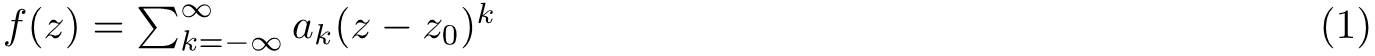Clearly if we want f(z0) is zero we require ak is zero for k smaller and equals to zero. Consequently we define that z0 is a zero of order n if: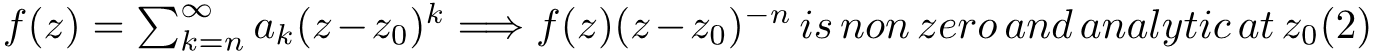We can observe some useful properties from (2). Firstly, an n order zero implies that up to the (n-1)th derivative of f(z) are also zero at z0 and vice versa. We can use this property to determine the order of zeros of a function, in the case that they are not so obvious. Secondly, The zeros of f(z) are the poles of 1/f(z) for obvious reason, provided that f(z) is not identically zero. We would like to find the properties of the Laurent series of  1/f(z):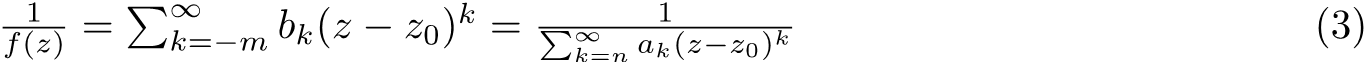The value of m can be determined by multiply the denominator of right hand side : We obtain a series that constantly equals to one. This requires m=n, and we see the coefficients bk are fixed by the values of ak .

In summary we found thatIn particular, if f(z) is analytic and non-zero at z, we now from (2) know that n=0 and thus from (4) 1/f(z) is also analytic and non-zero at z0.

### Introduction&Instructions

Hi, this is Cocteau. I hope this message finds you well.

Here, Cocteaupedia is a website where I store my thoughts and notes on Mathematics and Theoretical Physics. I like to imagine that the mind of an individual human is a little universe. Formulating my learning traces is my way of constructing this universe, and part of my ultimate goal is to understand the grand universe through the reflections of my little universe. What a cute thing!

Before Cocteaupedia was created, I was mostly building this universe on papers. However, due to my perfectionism I found that for me, it can be very hard to keep the note been taking, without starting to rubbishing them. As a result I frequently end my old plan and restart a new plan, and gradually I felt that this was neither meaningful nor eco-friendly (in terms of the amount of paper I have wasted).

Creating digital notes largely solves this issue, and a website is the optimal choice: it can be accessed at any spacetime, and is readily available to be shared and discussed. This is the story of why I created Cocteaupedia.

On this platform, every post is related to a specific question I’ve asked while learning maths and physics. For instance, the ideal of a post can be initiated when I was wondering the origin of Schrödinger’s  equation, or when I tried to think about how to calculate the residue of 1/ez+1 . I will try to organize them into topics and subtopics, displayed as Categories. For each post, important concepts that aren’t explained will be highlighted in bold. Only lengthy formulas will be written in LaTeX and displaced as pictures. This is due to display issues, which force me to write short, in-text mathematical relations or definitions – which are less necessary to be displayed as formulas – in regular text. Italic and bolded text will be used for emphasis.

There are, for now, no further instructions necessary to ensure smooth reading of the posts.

Thank you for reading this, I hope Cocteaupedia can be a good piece of work for you.

Cocteau

Created 20 May 2023

Modified 29 May 2023

[Homepage picture: Wikipedia-String Theory]

### 1. Weierstrass Approximation Theorem

We want to show that The set of polynomials is dense in the space of continuous functions. We shall firstly define the Bernstein Polynomials aswhere f is a continuous function with domain [0,1] and we can thus see that it is uniformly continuous.This means that in δ-ε language :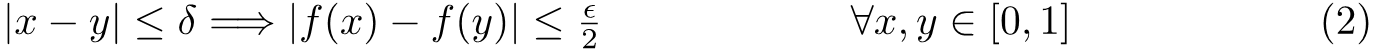here we manually set an interval in the domain. However, we are interested in the general relationship between two outputs without limitation ( other than the distance between two input must be in the range [0,1] ). So we want to now what happens when the |x -ξ| is larger than δ.

We recall the definition of norms in linear algebra. For finite vector space, the infinite norm is defined as: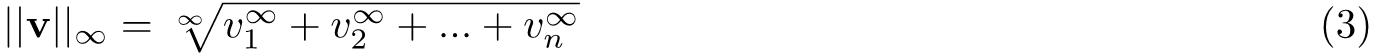It is easy to understand that this gives the largest entry (in terms of magnitude) of a vector. Note that this is true even if there exists more than one maximum entry. We expand this property into function space and define M to be the infinite norm of f(x). Then for |x -ξ| is larger than δ we write: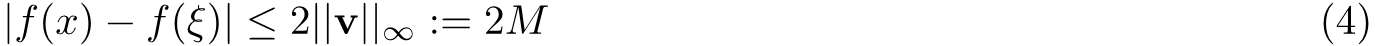Then combine (3) and (4) we obtain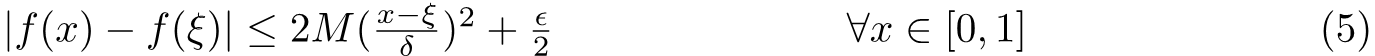We now want to use this relation to show that Bernstein polynomials can be used to approximate f(x). We notice that the Bernstein polynomial of f(ξ) is just f(ξ), where binomial expansion has been used to obtain this result. Then, using the fact that Bernstein polynomial is linear for f(x),In the second line above we putted the first term of the function into the expression of the polynomial and it came out will some calculation. Obviously, we need to set x=ξ to proceed and this yields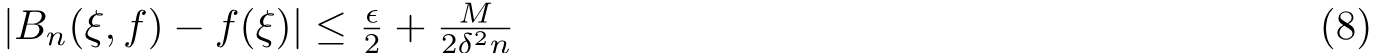What does this means? Remember that we have the freedom to make n as large as we want. This means that we can make the difference between Bernstein polynomials and our function as arbitrarily small as we want by approaching n to infinity.  i.e., Bernstein  polynomials converges to our f(x).

[Literature: Matt Young, MATH 328 Notes, Queen’s University at Kingston, 2006]

### 2. Taylor’s Theorem

Now we are ready to prove Taylor’s Theorem. Knowing polynomials span the space of continuous function, we now assume that the function is also infinitely differentiable. In this special case, instead of using Bernstein polynomials, we want to use the basis consists of 1,x,…,xn. Matching the value of our series and our function at a particular point yields the ordinary form of single variable Taylor series.

To be continued…

### 1. Derivative of a determinant

Consider the determinant W(t) of a n×n matrix Y which each element is a function of t. Assume elements to be independent variables. Then we could write: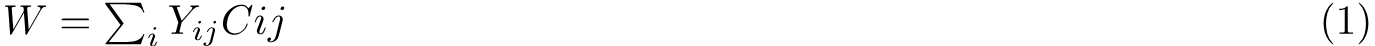Where Cij are the corresponding cofactors. Thus we have: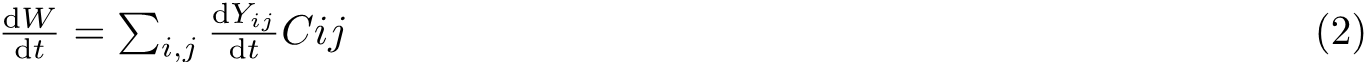Let’s define γi as the new matrices form by substituting the ith row with its derivative. Then we could write (2) in a tidier form: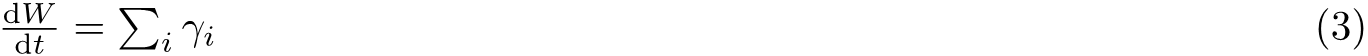### 2. Abel-Jacobi-Liouville identity

As we know, any system of linear ordinary equations can be extracted in to a single linear equation, namely: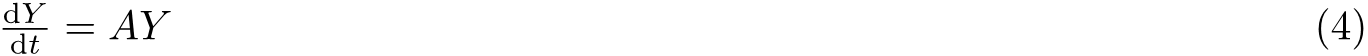And it is followed by that,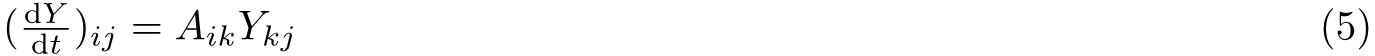So we obseverve that a particular row of the derivative is the linear combination of the original rows, since for the kth row of Y, different elements on jth column are multiplied by the same factor Aik. So of course, each term on the right hand side of (3) will be W times the corresponding element of Aii.
Thus,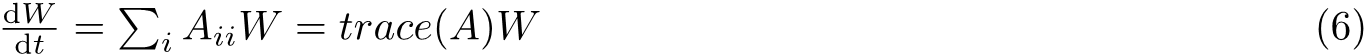This has resulted in some interesting conclusions. For example if the solutions are independent for any point within the domin, they must be independent entirely.

[Literature: Pontryagain 1962, Chapter 3]